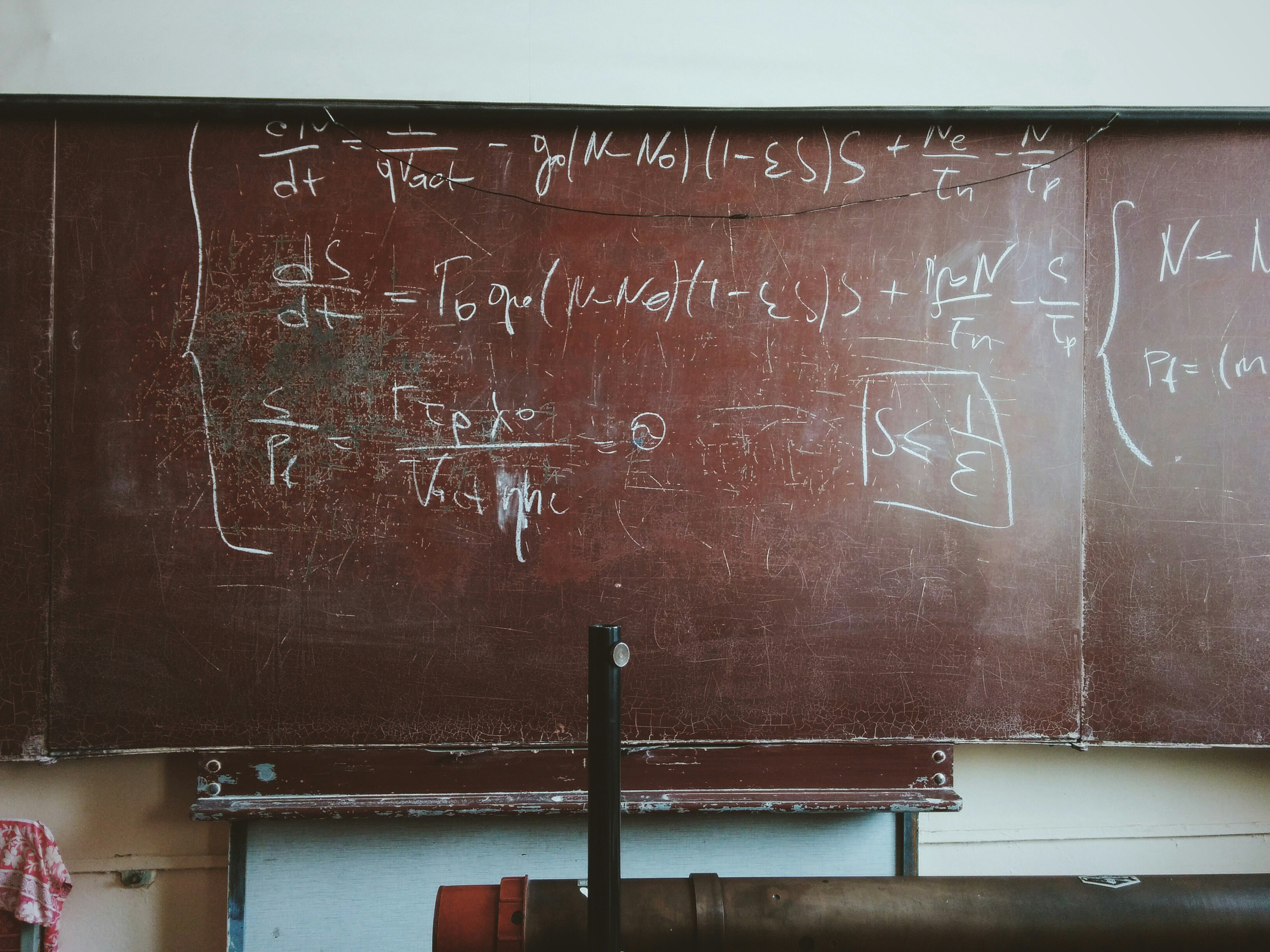# Quantitative Reasoning Aptitude Questions For NTS GAT, NAT Tests

## Most Important and Repeated Quantitative Reasoning Aptitude Questions For Competitive Exams

By ubaid ullahPublished 2 years ago 3 min read
LikePhoto by Roman Mager on Unsplash

In this article, I will go through some of the most important Aptitude questions with solutions for competitive exams such as the NTS GAT, NAT, PPSC, and others, and these questions based on quantitative reasoning. The following questions are most repeated in the NTS exam.

## First Question

If one-third of one-fourth of a number is 15, then three-tenths of that number is:

A. 35

B. 36

C. 45

D. 54

Solution

This question is related to the number system, here you have to first assume the number

Assume the number is x

Here one third means 1/3,

One forth means ¼

Three tenth means 3/10

Now to convert the statement to the mathematical equation it is

Then, 1/3 of 1/4of x = 15

1/3 × 1/4 x = 15

x = 15 x 12 = 180.

So, required number = 3/10 x 180 = 54.

## Second Question

Find the number, when 15 is subtracted from 7 times the number, the result is 10 more than twice the number

A. 5

B. 15

C. 7.5

D. 4

Solution

Again this question is of a number system, and you have to start by converting the statement to a mathematical equation. The equation is

Let the number be x.

7x -15 = 2x + 10

5x = 25

x = 5

## Third Question

The difference between a number and its three- fifth is 50. What is the number?

A. 75

B. 100

C. 125

D. None

Solution

To convert the question to the mathematical equation, first assume the required number is x

Then, x-3/5x = 50

2/5x = 50

x = (50*5/2) = 125.

## Fourth Question

The cost of renting a bike at the local bike shop can be represented by the equation Y = 2x + 2 where Y is the total cost and x is the number of hours the bike is rented. Which of the following ordered pairs would be the possible number of hours rented, x, and the corresponding total cost, y.

A. (0, -2)

B. (2, 6)

C. (6, 2)

D. (-2, -6)

Solution

Choice A and D should be omitted because the negative values should not make sense for this problem using time and cost. Choice B substitute would be 6 =2(2) +2 which simplified to 6 = 6. The coordinates in choice C are reversed from choice B and will not work if substituted for x and y. So here B option is correct.

## Fifth Question

Find the average of the first 97 natural numbers.

A. 47

B. 37

C. 48

D. 49

Solution

To find the sum of first n natural numbers, you have to use the formula

n(n+1)/2

Now here, the required is average of the first 97 natural numbers. So you have to divide the n(n+1)/2 by n which is 97 in this case.

= (97(97+1)/2)/97

= 49

## Sixth Question

Waqar has a bag of gumdrops. If he eats 2 of his gumdrops, he will have between 2 and 6 of them left. Which of the following represents how many gumdrops, x, was originally in his bag?

A. 4<X<8

B. 0<X<4

C. 0>X>4

D. 4>X>8

Solution

This problem is an example of a compound inequality, where there is more than one inequality in the question. In order to solve it, let x = the total amount of gumdrops will have. Set up the compound inequality, and then solve it as two separate inequalities.

Therefore the second sentence in the problem can be written as: 2< x<-2 and x-2<6. Add 2 to both sides of both inequalities: 2+2<x-2+2 and x-2+2<6+2; simplify: 4<x and x<8. If x is greater than four less than eight it means that the solution is between 4 and 8. This can be shortened to:4<x<8.

More Quantitative Reasoning Aptitude Questions With Solution For Aspirants

How To Solve Analogies Verbal Reasoning Questions.

Sharing Is Caring, Share It If you Learn Something New From It.

student
Like

### ubaid ullah

An Engineer, Freelancer, and content writer.

How does it work?

There are no comments for this story

Be the first to respond and start the conversation.